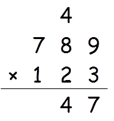This method is for multiplying two three digit number.

Let us say the numbers are ABC and DEF.

Write them as shown below.

A  B  C
D  E  F

Step 1: C × F

Step 2: (B × F) + (C ×E)

Step 3: (A × F) + (C × D) + (B × E)

Step 4: (A × E) + (B × D)

Step 5: A × D

Let’s take an example to understand this method.

789 × 123

Step 1: 3 × 9 = 27Step 2: (8×3) + (9×2) = 42 + 2(Carry) = 44Step 3: (7 × 3) + (1 × 9) + (8 × 2) = 46 + 4(Carry) = 50Step 4: (7 × 2) + (8 × 1) = 22 + 5(Carry) = 27Step 5: 7 × 1 = 7 + 2(Carry) =9So 789 × 123 = 97047